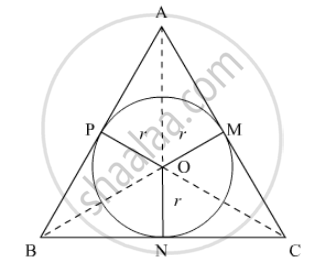Advertisement Remove all ads

# The Area of the Incircle of an Equilateral Triangle of Side 42 Cm is - Mathematics

MCQ

The area of the incircle of an equilateral triangle of side 42 cm is

#### Options

• $22\sqrt{3} c m^2$

•  231 cm2

• 462 cm2

• 924 cm2

Advertisement Remove all ads

#### Solution

Let ABC be the equilateral triangle such that AB = BC = CA = 42 cm. Also, let O be the centre and r be the radius of its incircle.AB, BC and CA are tangents to the circle at M, N and P.

∴ OM=ON=OP=r

Area of ΔABC = Area (ΔOAB) + Area (ΔOBC) + Area (ΔOCA)

⇒ sqrt3/4(42)^2=1/2xxrxxAB+1/2xxrxxBC+1/2xxrxx CA

⇒ sqrt3/4xx42xx42=1/2 r(AB+BC+CA)

⇒ 441sqrt3=1/2xxrxx(42+42+42)

⇒441sqrt3=1/2xxrxx(126)

⇒441sqrt3=63r

⇒r=(441sqrt3)/63

⇒ r=7sqrt3 cm

Area of the circle = pir^2=22/7 (7sqrt3)^2=22/7xx147=462 cm^2

Is there an error in this question or solution?
Advertisement Remove all ads

#### APPEARS IN

RD Sharma Class 10 Maths
Chapter 13 Areas Related to Circles
Q 9 | Page 69
Advertisement Remove all ads

#### Video TutorialsVIEW ALL 

Advertisement Remove all ads
Share
Notifications

View all notifications

Forgot password?
Course